Math resources Geometry Surface area

Surface area of a rectangular prism

# Surface area of a rectangular prism

Here you will learn about the surface area of a rectangular prism and how to calculate it.

Students will first learn about the surface area of a rectangular prism as part of geometry in 6th grade.

## What is the surface area of a rectangular prism?

The surface area of a rectangular prism is the total area of all of the faces of a rectangular prism.

Rectangular prisms have three pairs of identical faces – top and bottom, front and back, and left and right. Notice that it is always the opposite faces that are identical. Rectangular prisms are also known as cuboids.

To calculate the total surface area of a rectangular prism, calculate the area of each rectangular face and add them all together.

For example,

You can also use the net of a rectangular prism to find the surface area. The rectangular prism above unfolded into its net would be:

Surface area is measured in square units (e.g. mm^2, cm^2, m^2 etc).

### What is the surface area of a rectangular prism?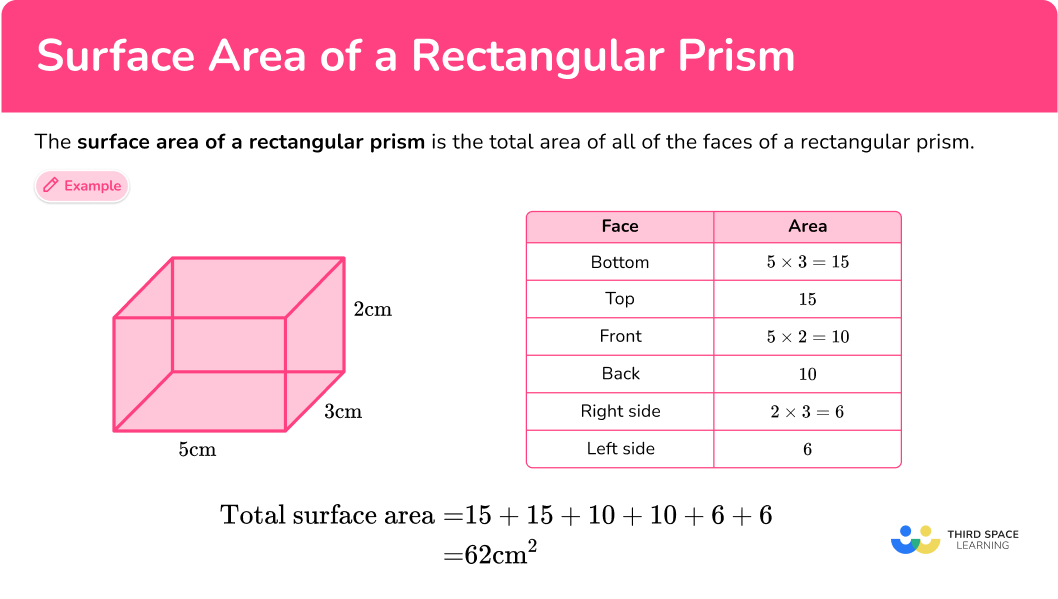## Common Core State Standards

How does this relate to 6th grade math?

• Grade 6 – Geometry (6.G.A.4)
Represent three-dimensional figures using nets made up of rectangles and triangles, and use the nets to find the surface area of these figures. Apply these techniques in the context of solving real-world and mathematical problems.

## How to calculate the surface area of a rectangular prism

In order to work out the surface area of a rectangular prism:

1. Calculate the area of each face.
2. Add the six areas together.
3. Include the units.

## Surface area of a rectangular prism examples

### Example 1: surface area of a rectangular prism

Calculate the surface area of the rectangular prism.

1. Calculate the area of each face.

The area of the bottom is 9 \times 3=27 \, cm^2.

The top face is the same as the bottom face, so the area of the top is also 27 \, cm^2.

The area of the front is 9 \times 4=36 \, cm^2.

The back face is the same as the front face, so the area of the back is also 36 \, cm^2.

The area of the right hand side is 3 \times 4=12 \, cm^2.

The left side face is the same as the right side face, so the area of the left side is also 12 \, cm^2.

It will make our work clearer if you use a table:

Total surface area: 27+27+36+36+12+12=150

3Include the units.

The measurements on the rectangular prism are in cm, so the total surface area of the rectangular prism is 150 \mathrm{~cm}^2.

### Example 2: surface area of a rectangular prism

Calculate the surface area of the rectangular prism.

Total surface area: 18+18+54+54+27+27=198

The measurements on the rectangular prism are in mm, so the total surface area of the rectangular prism is 198 \, mm^2.

### Example 3: surface area of a cube

Calculate the surface area of this cube.

Each face of a cube is the same. The faces are all squares. For this cube, the area of each face is:

8 \times 8=64

Since the cube has 6 equal faces, the area of one face can be multiplied by 6 to find the total surface area:

6 \times 64=384

The measurements on the cube are in cm, so the total surface area of the cube is 384 \mathrm{~cm}^2.

### Example 4: surface area with different units

Given that the base of this rectangular prism is the bottom rectangle, calculate the lateral surface area.

Notice that one of the measurements is in meters and the rest are in centimeters. Before you can calculate any areas, ensure all units are the same.

In this case, 0.1 \, m = 10 \, cm, so you can use 10 \, cm.

For the lateral surface area of a rectangular prism, do not include the base area (bottom and top).

Total surface area of the four lateral faces: 20+20+25+25=90

The measurements you have used are in cm, so the total lateral surface area is 90 \mathrm{~cm}^2.

### Example 5: surface area with a missing length

The surface area of this rectangular prism is 328 \mathrm{~cm}^2. Find the missing length.

Use the variable x to represent the missing side.

Total surface area: 14x+14x+84+84+6x+6x = 40x+168

Since the final surface area is 328 \, cm^2, you can use the equation to solve for the missing side:

40x+168=328

The missing side length is the value for x that makes the equation true.

One way to solve is by using substitution.

Let’s solve the equation for x=2.

\begin{aligned} & 40 \times 2+168=328 \\\\ & 80+168=328 \\\\ & 248=328 \end{aligned}

This is NOT true, so x 2.

Since x=2 was too small, let’s try x=4.

\begin{aligned} & 40 \times 4+168=328 \\\\ & 160+168=328 \\\\ & 328=328 \end{aligned}

This is true, so x=4.

The missing measurement is not the area, it is a side length, which is measured in singular units.

The width of this rectangular prism is 4 \mathrm{~cm}.

### Example 6: surface area word problem – missing height

Helena was making rectangular boxes out of wood. If she used 200 \mathrm{~cm}^2 of wood for the rectangular box shown above, what is the missing height?

You can unfold the rectangular prism, and use the net to find the area of each face:

The base is a rectangle that measures 2 \, cm by 6 \, cm. The area of each base is 12 \, cm^2.

Total area of the bases: 12+12=24

Subtract the area of the bases from the total amount of wood that Helena used, to see how much was used on the lateral faces: 200-24=176

The total area of the faces left is 176 \mathrm{~cm}^2.

Remember that the edges in a prism are always equal, so if you were to fold up the net, the 2 \, cm and 6 \, cm sides of the rectangle would combine to form an edge with each corresponding rectangle – making their lengths equal.

Labeling the missing length as x, this leaves the area of 4 faces:

• 6 \times x or 6 x
• 2 \times x or 2 x
• 6 \times x or 6 x
• 2 \times x or 2 x

Together they equal 176 \mathrm{~cm}^2, so the total of the missing areas can be written as:

6 x+2 x+6 x+2 x=176

or

16 x=176

Since 16 \times 11=176, the missing side length is 11.

The missing measurement is not the area, it is a side length, which is measured in singular units.

The missing side length is 11 \, cm.

### Teaching tips for the surface area of a rectangular prism

• Provide opportunities that connect the surface area of rectangular prisms to real world applications. The connection to wrapping gifts or painting the sides of a prism are popular examples. Also allow students to think critically about each real life connection.

Asking questions like “When wrapping a gift, is it possible to use more paper than the surface area? Why or why not?” or “When painting a rectangular prism in the real world, what is a situation where you might not paint the entire surface area?”

• Worksheets can be a useful practice tool for this topic, but make sure that students also have a sufficient amount of practice with real world 3D shapes and nets. Solving problems on worksheets require students to be able to work with 2D representations of 3D shapes.

This is much easier for students if they have worked extensively with these shapes in the real world and can easily picture and rotate them in their minds.

### Easy mistakes to make

• Calculating volume instead of surface area
Volume and surface area are different things – volume is the space within the shape and is measured in cubic units, whereas surface area is the total area of the faces and is measured in square units. To find surface area, you need to work out the area of each face and add them together.

• Thinking all lateral faces are equal
Only opposite sides are equal in a rectangular prism. This means that many rectangular prisms have 3 different sets of congruent rectangles.
For example,

It is possible for all lateral faces to be equal, but only if the base is a square.
For example,

### Practice surface area of a rectangular prism questions

1. Calculate the surface area of the rectangular prism.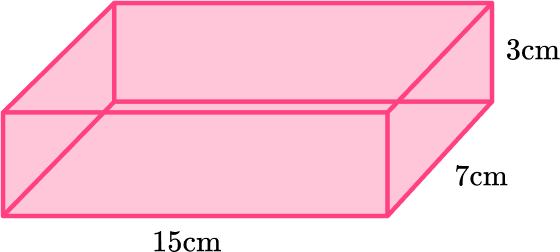342 \mathrm{~cm}^{2}315 \mathrm{~cm}^{2}222 \mathrm{~cm}^{2}462 \mathrm{~cm}^{2}Calculate the area of each of the six faces: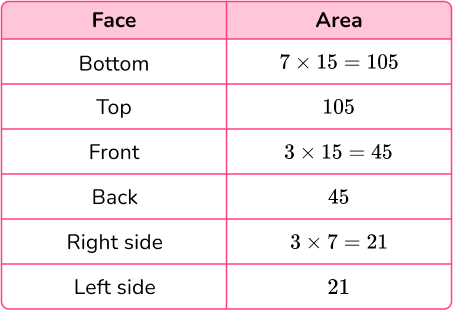Total surface area: 105+105+45+45+21+21=342\mathrm{~cm}^{2}

Since the dimensions are in centimeters, the surface area is in square centimeters.

2. The perimeter of the base is 16 inches. Calculate the surface area of the cube.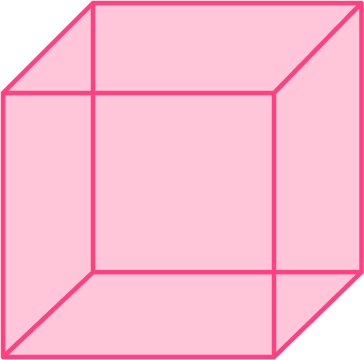64\mathrm{~inches}^{2}24\mathrm{~inches}^{2}96 \mathrm{~inches}^{2}256 \mathrm{~inches}^{2}The base of a cube is a square and all sides are equal.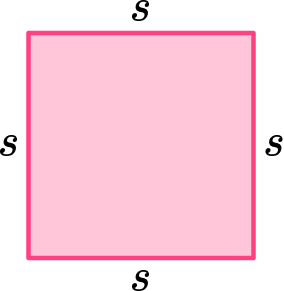Since the perimeter is found by adding all the sides together, it can be shown as s + s + s + s =16.

The side length of 4 makes this equation true, so each side is 4 inches.

Since it is a cube, all of the faces are the same square.

The area of each face is 4\times 4=16 \mathrm{~inches}^{2}.

There are six identical faces, so the total surface area of the cube is 6 \times 16=96 \mathrm{~inches}^{2}.

Since the dimensions are in inches, the surface area is in square inches.

3. Calculate the surface area of the rectangular prism.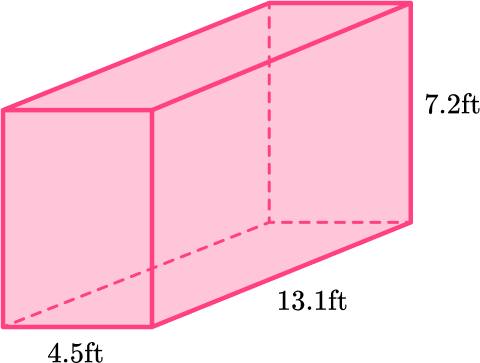247.5 \mathrm{~ft}^2495.18 \mathrm{~ft}^2371.34 \mathrm{~ft}^2185.7 \mathrm{~ft}^2Calculate the area of each of the six faces: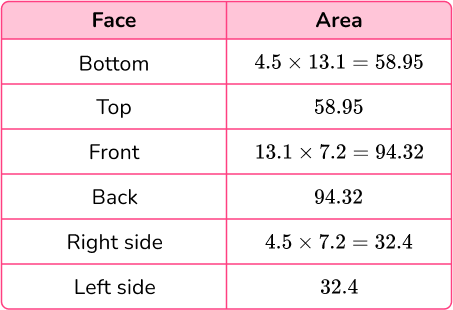Total surface area:

58.95+58.95+94.32+94.32+32.4+32.4=371.34 \, ft^2.

4. Calculate the surface area of this rectangular prism.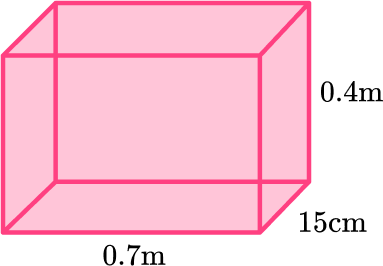33.56\mathrm{~cm}^{2}4.2 \mathrm{~cm}^{2}12,400 \mathrm{~cm}^{2}8,900 \mathrm{~cm}^{2}Some of the measurements are in m and one is in cm.

All of the measurements need to be in the same units, so convert the meters to centimeters.

0.7 \, m=70 \,cm and 0.4 \, m=40 \, cm.

Now calculate the areas: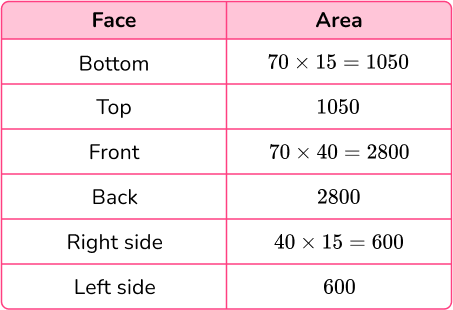Total surface area:

1,050+1,050+2,800+2,800+600+600=8,900\mathrm{~cm}^{2}.

5. Given that the surface area of this rectangular prism is 142 \, \mathrm{~cm}^{2}, find the value of x.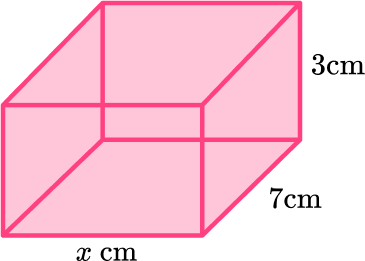6.76 \mathrm{~cm}14.2 \mathrm{~cm}20.29 \mathrm{~cm}5 \mathrm{~cm}Calculate the area of each of the six faces: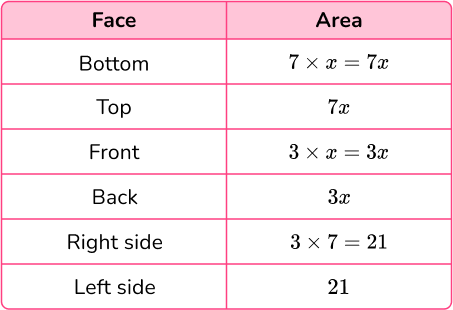Total surface area: 7x+7x+3x+3x+21+21=20x+42.

Since you know the total surface area is 142\mathrm{~cm}^{2}, the equation 20 x+42=142 can be used to find the height.

Solve this equation by substituting in the answer choices:

\begin{aligned} & 20 \times 6.76+42=142 \\\\ & 135.2+42=142 \\\\ & 177.2=142 \end{aligned}

This equation is not true.

Since 177.2 is larger than 142, \, x needs to be a smaller value.

The only answer choice that is smaller is 5.

\begin{aligned} & 20 \times 5+42=142 \\\\ & 100+42=142 \\\\ & 142=142 \end{aligned}

This equation is true.

The missing measurement on the base is 5 \mathrm{~cm}.

6. A pet store covers the left side, right side and back side of aquariums with blue paper that looks like water. Below are the measurements of the small and large tank. How much more paper does the large tank use than the small?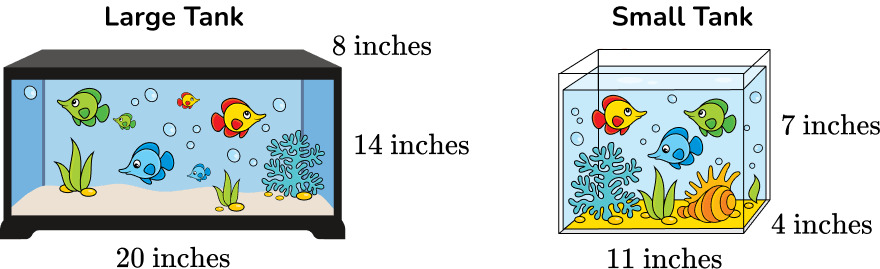133 \text { inches}^2504 \text { inches}^2371 \text { inches}^2806 \text { inches}^2Calculate the area of each of the left, right and back faces for each aquarium:

Large Aquarium;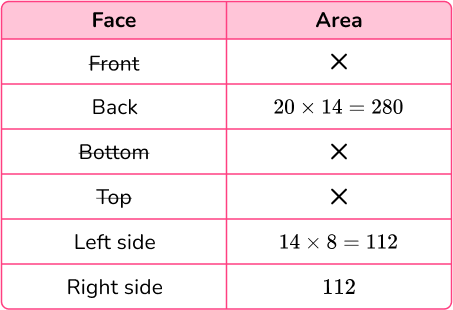Surface area of the blue paper used on the large aquarium:

280+112+112=504 \text { inches}^2

Small Aquarium;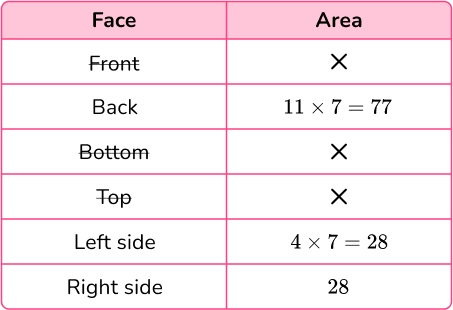Surface area of the blue paper used on the small aquarium:

77+28+28=133 \text { inches}^2

Subtract to find the difference:

504-133=371 \text { inches}^2

## Surface area of a rectangular prism FAQs

What is a cuboid?

It is the name for a three-dimensional shape that is made up of rectangles and/or squares. A cuboid is another name for rectangular prisms.

What is the difference between a rectangular prism and a rectangular pyramid?

They both have a rectangular base, but a pyramid has one base and triangular lateral faces that meet in a point. A rectangular prism has two bases and rectangular lateral faces.

Is there a surface area of a rectangular prism formula?

Yes, the general formula is 2(lw+wh+hl).

How is the volume of a rectangular prism calculated?

The volume can be calculated with the formula V=l \times w \times h.

## Still stuck?

At Third Space Learning, we specialize in helping teachers and school leaders to provide personalized math support for more of their students through high-quality, online one-on-one math tutoring delivered by subject experts.

Each week, our tutors support thousands of students who are at risk of not meeting their grade-level expectations, and help accelerate their progress and boost their confidence.